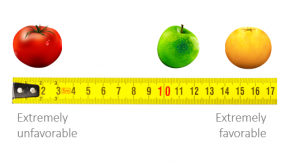# 2.1 Introduction

### MeasurementMeasurement – assigning numbers or other symbols to characteristics of objects according to certain pre-specified rule

– one-to-one correspondence between the numbers and characteristics being measured

– the rules for assigning numbers should be standardized and applied uniformly

– rules must not change over objects or time

### ScalingScaling – involves creating a continuum upon which measured objects are located.

### Primary Scales of Measurement

Nominal:

• numbers serve as labels for identifying and classifying objects
• not continuos

Ordinal:

• numbers indicate the relative positions of objects
• but not the magnitude of difference between them

Interval:

• differences between objects can be compared
• zero point is arbitrary

Ratio a.k.a. metric:

• zero point is fixed
• ratios of scale values can be computed

### Primary Scales of Measurement

 Scale Basic Characteristics Common Examples Marketing Examples Permissible Statistics Descriptive Inferential Nominal Numbers identify and classify objects Social security numbers, numbering of football players Brand numbers, store types sex, classification Percentages, mode Chi-square, binomial test Ordinal Numbers indicate the relative positions of the objects but not the magnitude of differences between them Quality rankings, ranking of teams in tournament Preference rankings, market position, social class Percentile, median Rank-order correlation, Friedman ANOVA Interval Differences between objects can be compared; zero point is arbitrary Temperature (Fahrenheit, Centigrade) Attitudes, opinions, index numbers Range, mean, standard deviation Product-moment correlations, t-tests, ANOVA, regression, factor analysis Ratio Zero point is fixed; ratios of scale values can be compared Length, weight, time, money Age, income, costs, sales, market shares Geometric mean, harmonic mean Coefficient of variation

### Comparison of Scaling Techniques

Comparative Scales:

• involve the direct comparison of stimulus objects.
• data must be interpreted in relative terms
• have only ordinal and rank-order properties

Non-comparative Scales:

• each object is scaled independently
• resulting data is generally assumed to be interval or ratio scaled

– nature of the research

– variability in the population

– statistical considerations